## FLARE On Challenge (2015) #10

This challenge contains a large Windows Portable Executable File which is nearly 3.4 MB. It is usually difficult to reverse engineering such a large file. However, if you have noticed the special resource embedded into this file, things will become easier: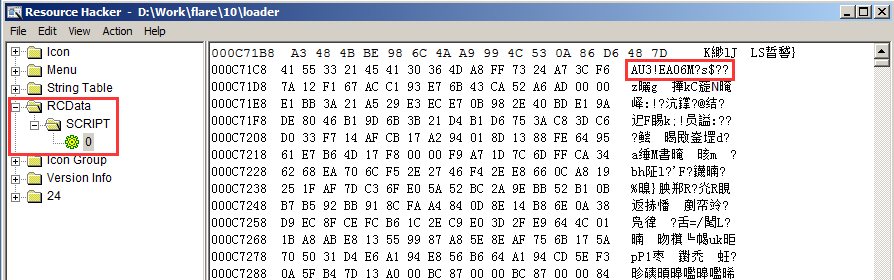This file is actually a compiled AutoIT program and we can extract the embedded AutoIT script with many tools like exe2aut. With the help of exe2aut I have extracted one AutoIT script and three executable files:
``` File Name: ioctl.exe Size: 46,080 bytes MD5: 205af3831459df9b7fb8d7f66e60884e SHA1: dfb2dc09eb381f33c456bae0d26cf28d9fc332e0 SHA256: 44473274ab947e3921755ff90411c5c6c70c186f6b9d479f1587bea0fc122940```
``` File Name: challenge-xp.sys Size: 2,688,640 bytes MD5: 399a3eeb0a8a2748ec760f8f666a87d0 SHA1: 393f2aefa862701642f566cdaee01a278e2378c0 SHA256: 57b1d7358d6af4e9d683cf004e1bd59ddac6047e3f5f787c57fea8f60eb0a92b```
``` File Name: challenge-7.sys Size: 2,689,536 bytes MD5: dade1de693143d9ef28fe7ebe7a7fb22 SHA1: 745ba710cf5d4a8cbb906f84e6096ca1b9a1bae3 SHA256: 59dbf937021c7856bad4155440dbd2e0c5422f06589bb11e5ac0b3300aad629c ```
Apparently, there are two driver files, one is for Windows XP and the other one is for Windows 7. And the ioctl.exe, from the file name, we can guess that it is used to communicate with the driver.

Let’s see what will the AutoIT script do at first. The AutoIT script contains a series of functions used to manage the service like _startservice(), _stopservice(), _serviceexists(), _servicerunning(), _createservice(), _deleteservice(), and the core logic is shown as below: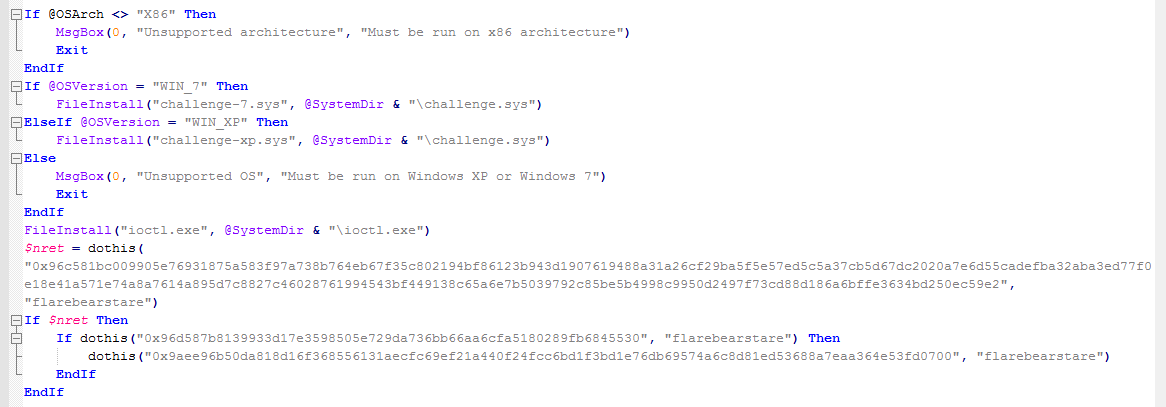Firstly, it will check if the system architecture is x86, if so it will drop a driver file to the system directory based on the OS version, after this it will also drop the ioctl.exe to the system directory. Next, it executes the function dothis() with two arguments, one is a hex string and the other one is an ASCII string “flarebearstare”. If the return value of that function is true, it will execute the dothis() function again with a different hex string. If the second dothis() returns true, it will then execute the third dothis() function. So what does the dothis() function do?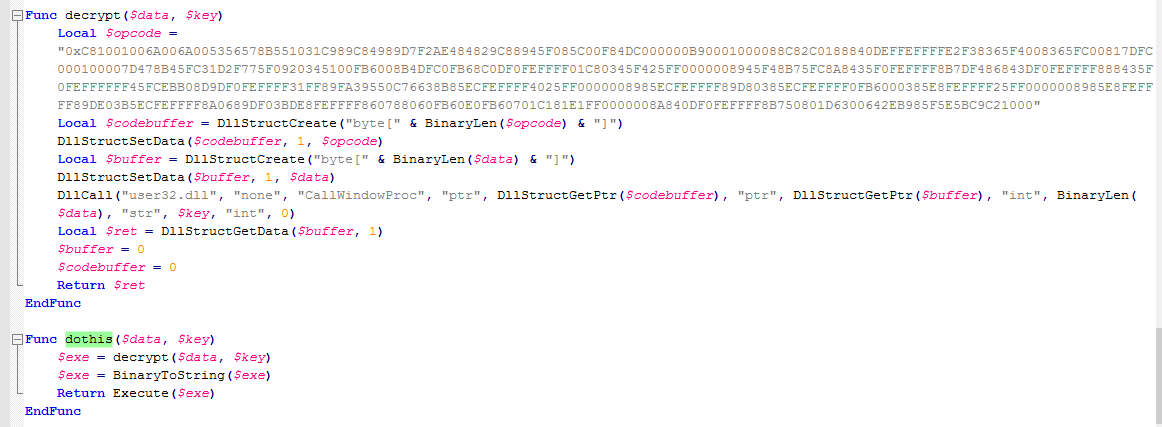The dothis() function actually receives two arguments: data and key. It will decrypt the data with the key and execute the decrypted data. In the decrypt() function, it will execute an opcode by calling API CallWindowProc() and pass the data and key as arguments.

As we already know that the API CallWindowProc() will be called, we can just set a breakpoint on this API in a debugger and follow the API to execute the opcode, and then get the decryption result. However, if you run this program in a debugger, you may probably receiver this error: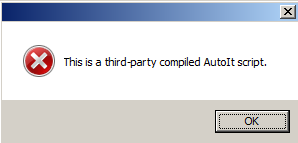What is going on here? Let’s search for the error string in IDA, and we can find the following piece of code: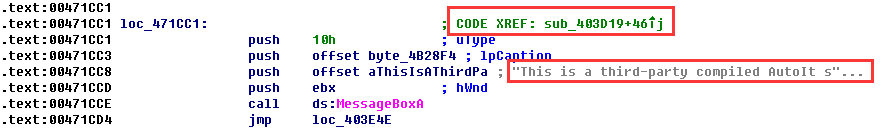If we follow the code reference here we can find the root cause: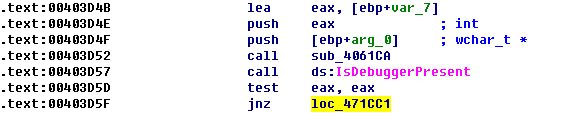There is an anti-debug check! Ok, to bypass this check we can just patch the “jnz” at 0x00403D5F to “jz”, and then continue the expectation. And finally, our breakpoint get hit in the debugger: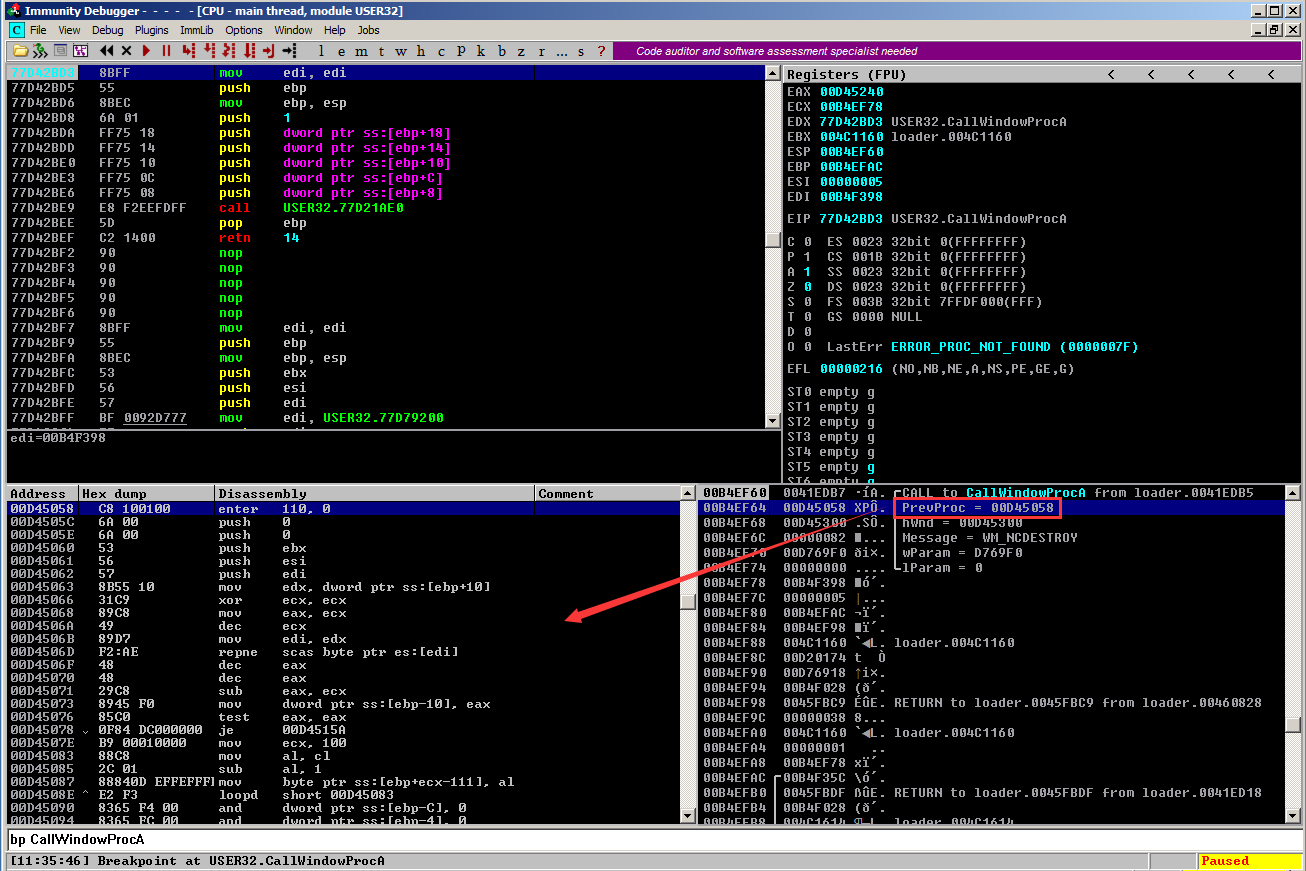The first arguments pass to the CallWindowProc() contains the address of the opcode, now we can put a breakpoint on the first instruction of the opcode and continue the execution. When the breakpoint on the opcode triggered, we can then execute to the “retn” instruction and here the decryption result can be found from the stack: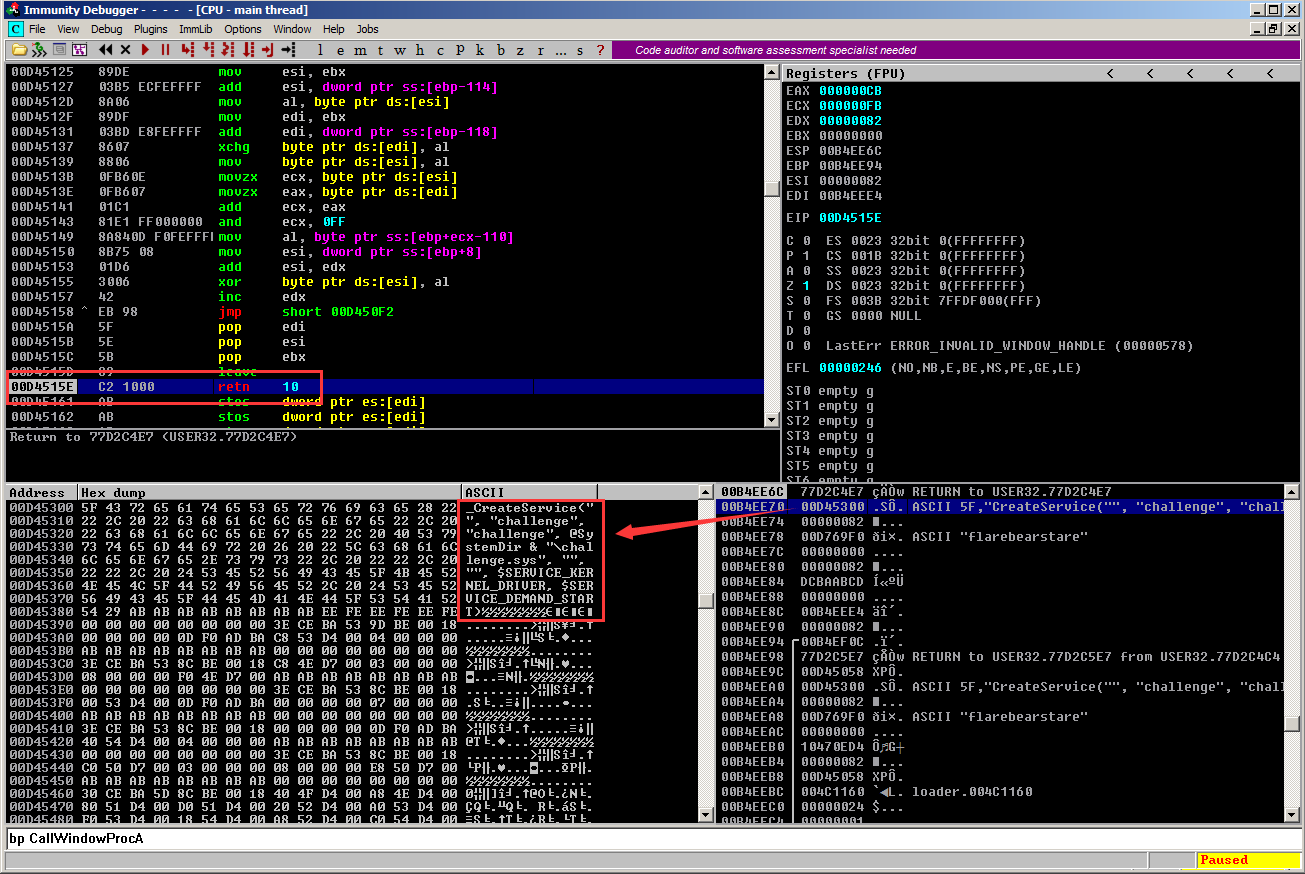Repeat the above process for the other two dothis() function, we can have all the decryption code:
``` _CreateService("", "challenge", "challenge", @SystemDir & "\challenge.sys", "","", \$SERVICE_KERNEL_DRIVER, \$SERVICE_DEMAND_START) _StartService("", "challenge") ShellExecute(@SystemDir & "\ioctl.exe", "22E0DC") ```
From those codes we know that the driver dropped by this program is registered as a service named “challenge” and then an IoControlCode 0x22E0DC is send to this driver through ioctl.exe.

Now it is the time to analyze the driver. As only one of the driver will be chosen (based on the OS version), so the two drivers must have same functionality and we only need look at one of them. I chose the one for Windows 7. The Entry Pointer of this driver seems normal, it will create a device named “challenge” and then register the unload routine and the dispatch routines. All the dispatch routines are pointing to a same function located at address 0x0029CD20, and if you look at the Graph Overview of this function, you may see something like this: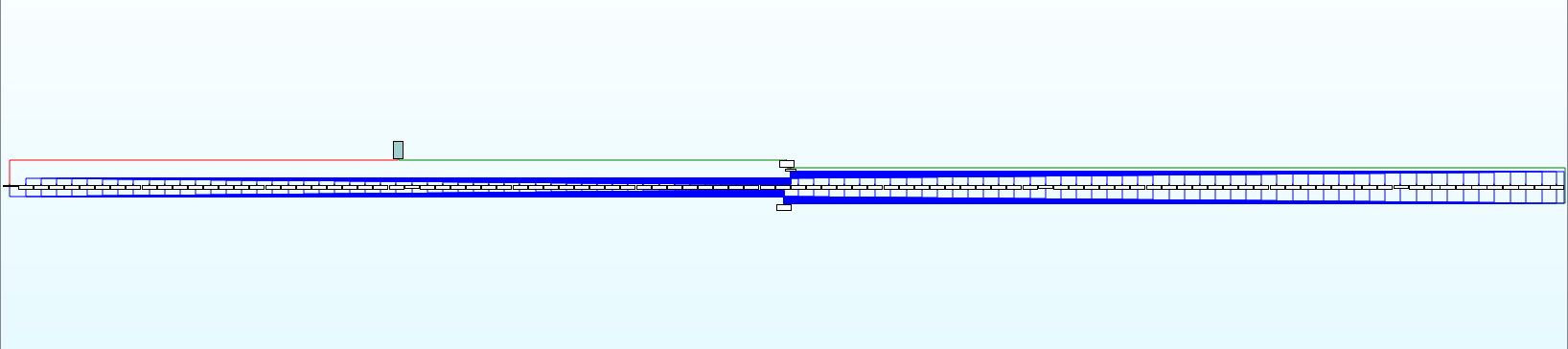Terrible, right? In the beginning of this function, it will retrieve the IoControlCode sent to this driver and sub it with 0x22E004, then it will use the result of the subtraction as an index to retrieve another index from a table located at address 0x0029D614, then the second index will be used to retrieve the jump destination address from a jump table located at address 0x0029D480: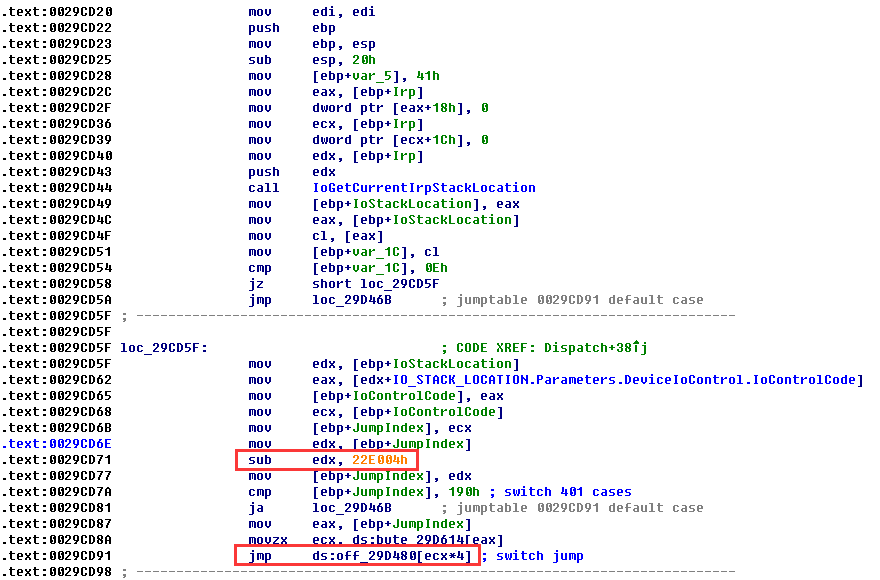So what is the first IoControlCode passed to this driver? Yes, it’s 0x22E0DC. So we can calculate the first index: 0x22E0DC – 0x22E004 = 0xD8, and the we can have the second index which is 0x36, and with the second index, we can finally found the jump address which should be 0x0029D180: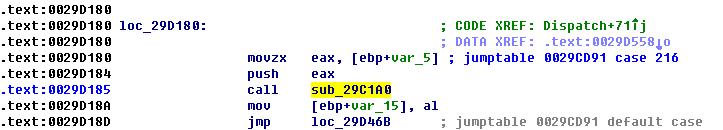Here it only calls another function located at 0x0029C1A0, and let’s move to this function. This function also looks terrible: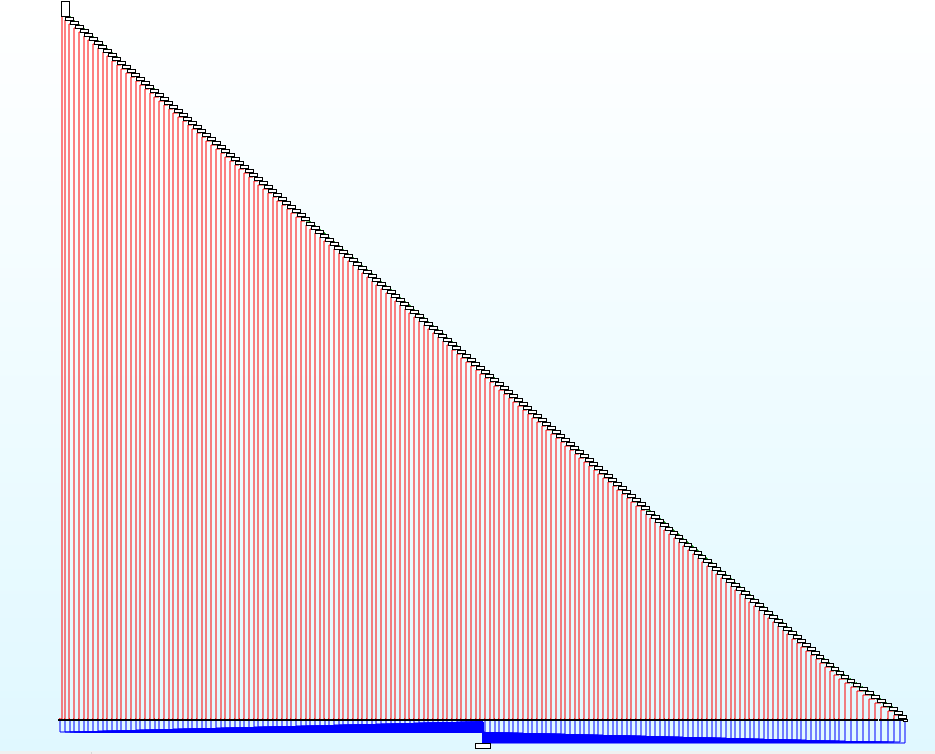But do not be panic, let’s look at this function in details:The functionality of this function is actually easy to understand, it will test each bit of a input sequence to see if that bit is 0 or 1, and then jump to different branch based on the test result. Basically, if all the bit test except the last one do not jump to the exit branch, we will get what we want. The following IDA Python script can be used to calculate the correct input sequence:

```from idaapi import *

def bit_reverse(c):
out = 0
for i in range(0, 8):
out <<= 1
c1 = c & 0x01
out |= c1
c >>= 1
return out

out = ''

c = 0
for i in range(0, 8):
c <<= 1
for j in range(0, 2):
c |= 0
c |= 1
else:
raise Exception('unknown instruction')
for j in range(0, 3):
else:
raise Exception('unknown instruction')
out += chr(bit_reverse(c))

print out
```

Ok, it asks us to try another IoControlCode 0x22E068. We can use the same way described before to calculate the jump destination and the function we should care about this time is located at address 0x0002D2E0, another terrible function: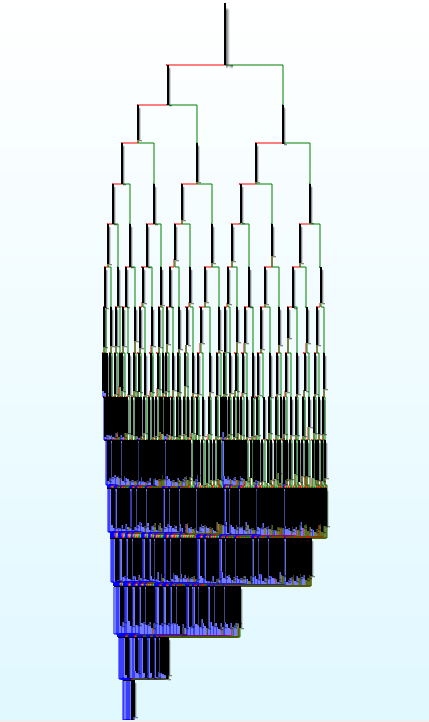There are a lot of branches in this function which makes it difficult for us to understand the logic. But one good thing is that no matter what code path the function will walk through, a call at the end of this function will be executed: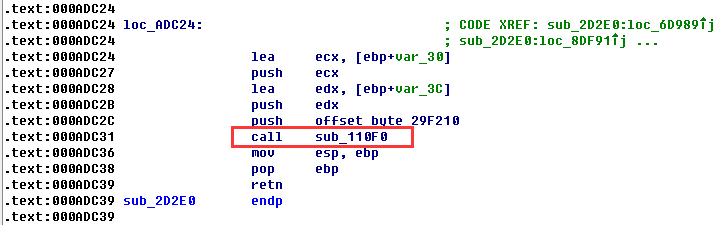This call received three arguments, and a quick analysis on it suggests that it is possibly uses the Tiny Encryption Algorithm (TEA) to decrypt some data. So how do we know what data will be decrypted? or what is the decryption result? I believe the easiest way to answer this is through debugging.

Let’s setup the kernel debugging environment at first since we are going to debug a driver. Here I use two machines, one is my physical machine (running Windows 8) and the other one is a Virtual Machine with Windows 7 installed. There is a wonderful tool named VirtualKD can make things easier. And there are a lot of tutorials online to teach you how to setup a kernel debugging environment, so I will not talk about it in details here, you may refer to the following article if you have not try this:
http://www.hexblog.com/?p=123

Now let’s set a breakpoint on the call at 0x000ADC31 and a breakpoint at the beginning of the dispatch routine at 0x0029CD20, then run the executable file of this challenge in the VM. If nothing goes wrong, we will stop at the dispatch routine like below: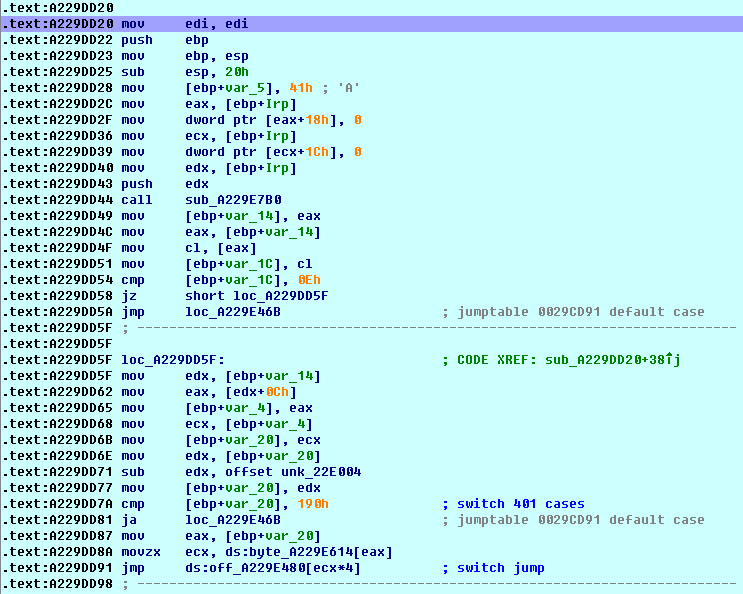Then we can just modify the IoControlCode to 0x22E068 (or use the ioctl.exe to issue this IoControlCode) and continue, and we will stop at the call we are interested: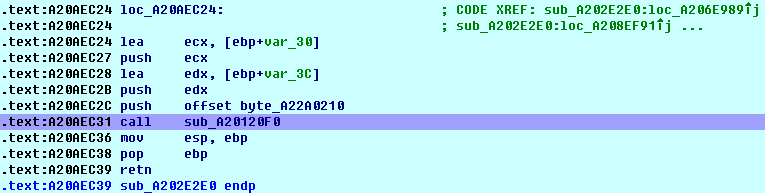However, after executes this function, we get nothing we want, the decrypted data seems meaningless. So what is the problem here? As this call aims at decrypting some data, let’s see what data is being decrypted. The data to be decrypted is passed as the first argument which should be a byte array, and if you look at this argument during debugging, you may be surprised that the array is all zeros when the decryption function is called, that is to say, the data to be decrypted is not initialized! Why?

If you look at the data reference of each byte in that array, you will find that each byte will be assigned a value by a separate function. However, those functions may not get executed when we reach the decryption function and that’s may be the reason why we have an empty array. Knowing this, we can try to initialize the array manually before we execute the decryption function. This can be done by the following IDA Python script:

```from idaapi import *

out = ''

for i in range(0, 0x28):

found = False

if GetMnem(ref) == 'mov':
for i in range(0, 2):
else:
raise Exception('unknown instruction')
found = True
break
else:
raise Exception('unknown instruction')

if found == False:
break

```

And this time the decyption function gives us what we want: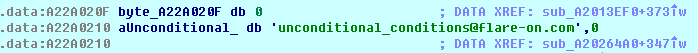Posted in CTF | Tagged , , , | Comments Off on FLARE On Challenge (2015) #10

## FLARE On Challenge (2015) #9

This challenge contains a Windows Portable Executable file in a very small size (4,608 bytes), typically, this kind of files is written in Assembly Lagrange. If you load this file into IDA, you may notice that the file is badly obfuscated and hard to analyze statically. But if you look at the Strings (Shift + F12), you may find some interesting code located at 0x00401000: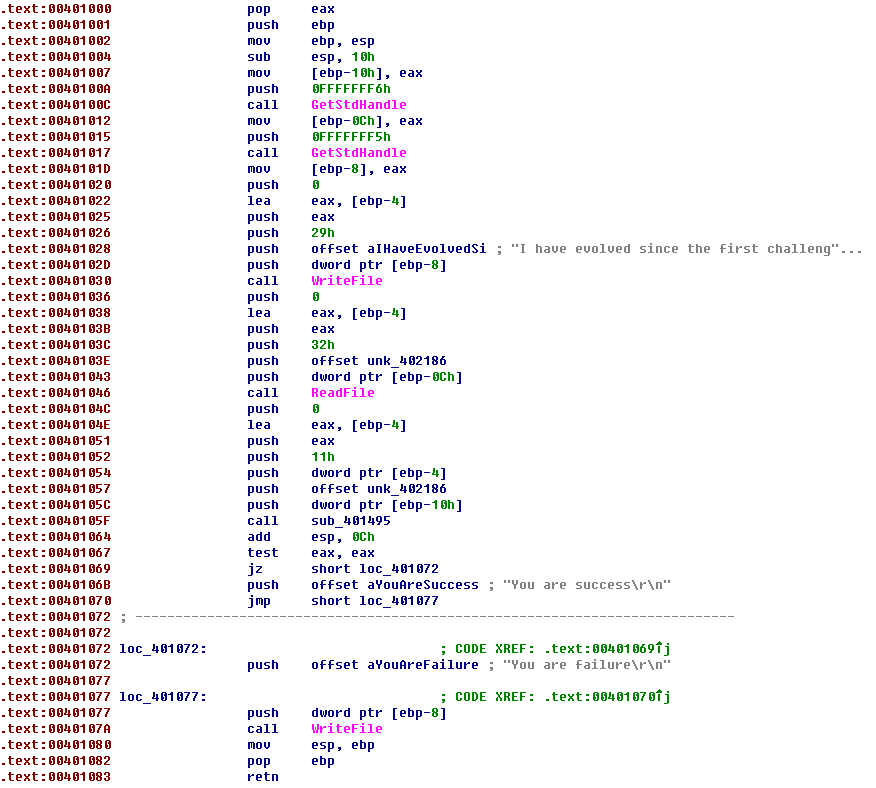Those code looks very similar to challenge #1 and challenge #2, so how about executing this program in a debugger and putting a breakpoint at 0x00401000? Unfortunately, the breakpoint here will not being hit. Eh… how about putting a breakpoint on the API it may calls like WriteFile() or ReadFile()? Badly, it seems cannot work either. But if you are not give up, you will finally find that a breakpoint on GetStdHandle() will eventually interrupt the execution of this program. That’s a good thing! Let’s execute this program step by step after hit the second GetStdHandle(). After several instructions, I am surprised that the program actually calls the API WriteFile():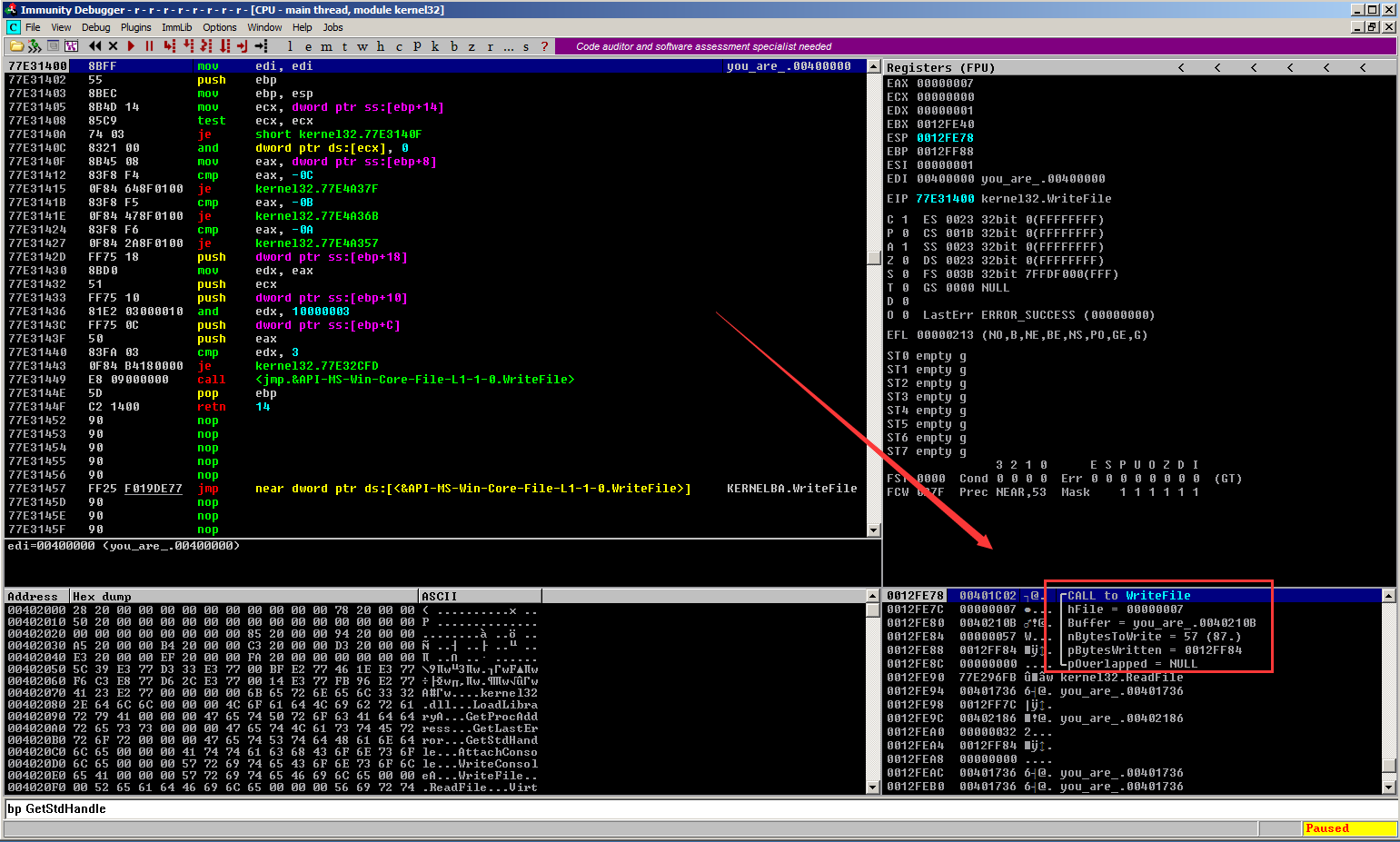So why our previous breakpoint on WriteFile() did not work? When I look at the breakpoints lists, I realized that it is because the debugger “play a joke” on me: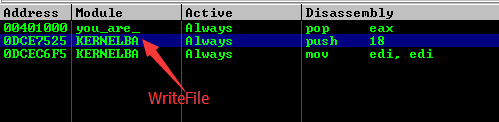The breakpoint here is set on the WriteFile() function from module Kernelbase.dll, however, the WriteFile() function being called at real time comes from module kernel32.dll: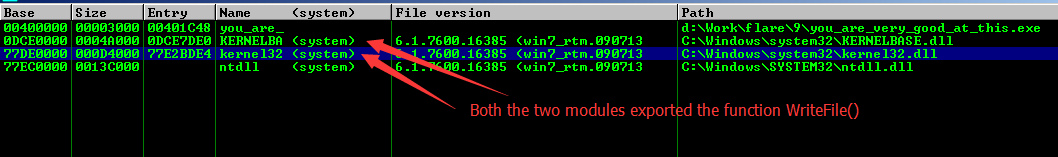Understand this, now we can put a breakpoint on the ReadFile() function in the kernel32.dll and it will hit as expected. After provides some fake input to the ReadFile() function, I continue executing this program on single steps. And finally, it comes to the interesting part: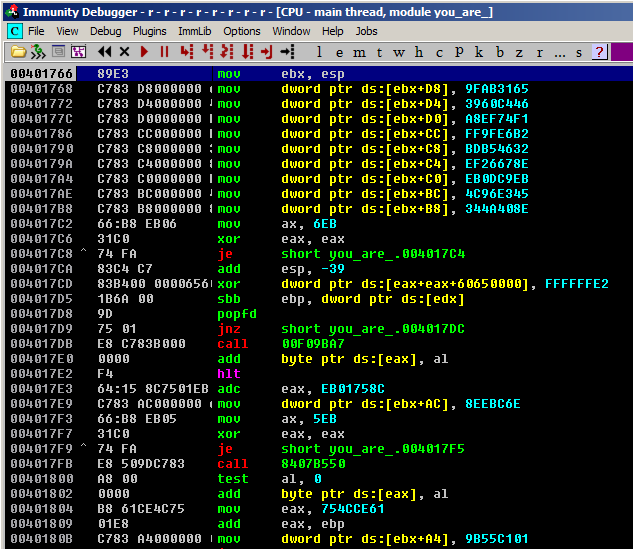If you execute the code from here step by step, you will find that although the code is badly obfuscated, the verification logic is easy to understand: firstly, it will initialize a table on the stack, and retrieve an index from this table, and then it will use this index to retrieve a second index from the same table. Next, it will use the second index to retrieve a byte from that table and XOR it with the relevant byte of our input data. After this, it will use the second index to retrieve another byte from that table and do a left rotation with the XOR result. And finally it will compare the rotate result with a third byte retrieved from that table.

If you cannot understand the algorithm clearly through my description, the following Python script may help:

```table  = '\xCC\xA9\x6D\x77\x0A\xF2\x4E\x58\xC1\x8A\x2F\x9F\xD8\x6C\x58\x43'
table += '\xF9\x1D\xC8\x92\x1F\x5C\xC3\x98\xC7\xF8\xD7\x03\xB0\x8A\x3C\xE7'
table += '\x1F\x1A\xCD\x02\x13\xF6\x07\x73\xF2\x33\xC8\xF0\xBA\xC2\xCC\xB8'
table += '\x80\xF0\x48\x77\xF9\x9A\x50\x55\xDC\x01\xE1\xEB\x16\x5A\x6B\xC6'
table += '\xC3\x0E\x8E\x27\x5D\xC3\xF4\x4E\x06\x42\xBC\xF2\x5D\x56\x30\x2E'
table += '\x19\x53\x53\x66\xD2\xF9\x03\xCF\x12\x06\x17\x1A\x0A\x0D\x02\x1E'
table += '\x16\x10\x1F\x09\x23\x0F\x14\x25\x1D\x03\x19\x21\x0B\x28\x13\x00'
table += '\x26\x08\x1B\x0C\x04\x27\x24\x1C\x01\x22\x18\x20\x11\x15\x05\x0E'
table += '\x07\x2A\x2B\x2C\xAC\xBE\xF5\x4E\x75\xBC\x87\xB8\x16\x67\x6B\x5C'
table += '\xFA\xF1\xF9\x93\xF2\xD4\xF8\x23\xB9\xC8\x11\x7E\xCA\x56\xD6\x1B'
table += '\x0A\xDA\x6E\xB5\x01\xC1\x55\x9B\xB8\x61\xCE\x4C\x6E\xBC\xEE\x08'
table += '\xF4\x64\x15\x8C\x65\x60\xE2\x1B\x8E\x40\x4A\x34\x45\xE3\x96\x4C'
table += '\xEB\xC9\x0D\xEB\x8E\x67\x26\xEF\x32\x46\xB5\xBD\xB2\xE6\x9F\xFF'
table += '\xF1\x74\xEF\xA8\x46\xC4\x60\x39\x65\x31\xAB\x9F\x01\x00\x00\x00'

def ror(n, c):
c = c % 8
t1 = (n >> c) & 0xff
t2 = (n << (8-c)) & 0xff
return t1 | t2

out = ''
for i in range(0, 41):
index1 = ord(table[i+0x58])
index2 = ord(table[index1+0x58])
ch1 = ord(table[index2+0xB0])
ch2 = ord(table[index2+0x84])
ch3 = ord(table[index2+0x2C])
out += chr(ror(ch3, ch2) ^ ch1)

print out
```

Run this script we can get the following result: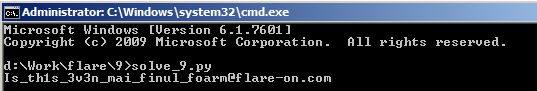Posted in CTF | Tagged , , , | Comments Off on FLARE On Challenge (2015) #9

## FLARE On Challenge (2015) #8

When you open this challenge directly in IDA, you may as disappointed as me, there is only a few code available at the Entry Point and they seems do nothing useful: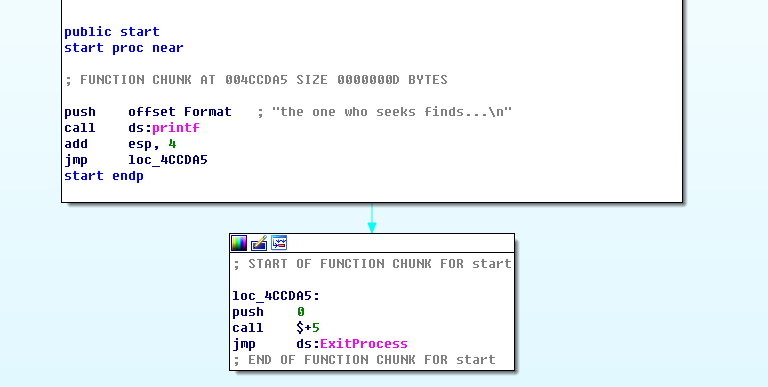However, if you open this file in a text editor, you may find the secret: there are a lot of Base64-like strings inside this file: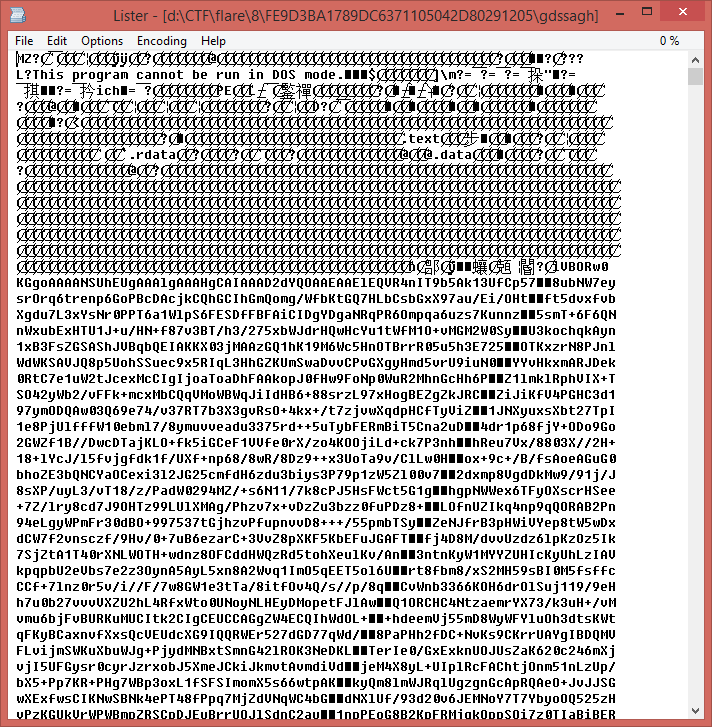After decodes those strings, you can have a PNG file looks like below:The first time I saw this picture, I believe that some information must be hidden into it. After Googling, I found a wonderful tool named Stegsolve which can help with analyzing the hidden information in a picture.

If you are not familiar with Steganography, I recommend you to read the following Wiki page:
https://en.wikipedia.org/wiki/Steganography

One famous approach to hide information into a picture is to store each bit of the information into the least significant bit (LSB) of the 8-bit color value of each pixel (24-bit bitmap). So let’s use the Stegsolve to extract this information, and the extracted data looks like below: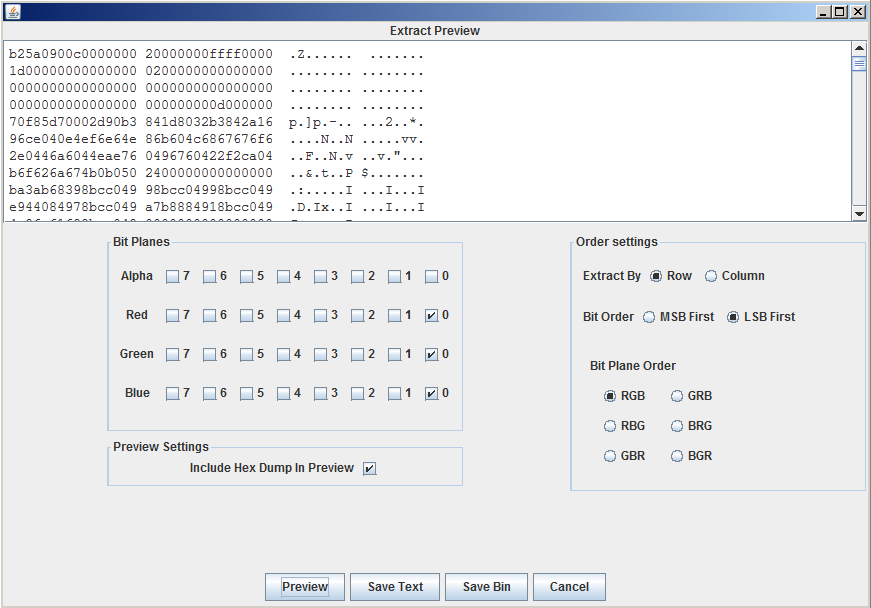At the first glance, there seems no useful inforamtion. However, if you are very familiar with the Windows Portable Executable file format, you may find some similarities if you compare the extracted binary data with a PE file: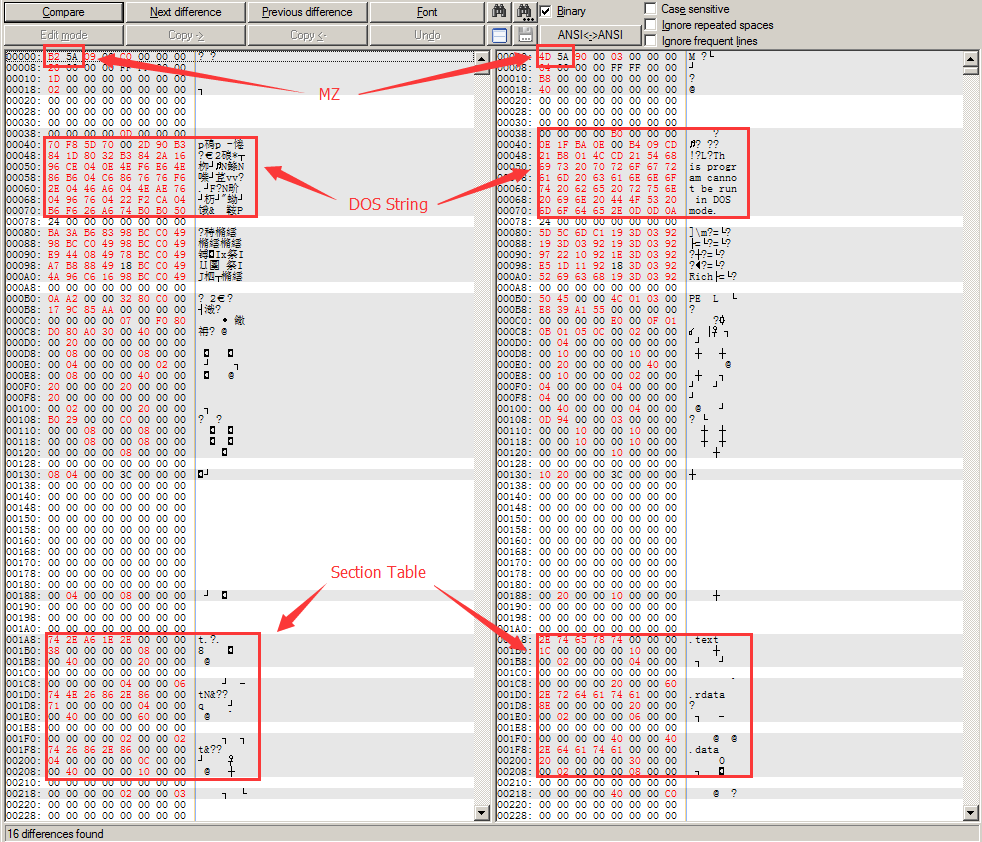Now we can guess that the information hidden in this picture is actually an encrypted PE file and let’s see if we can decrypted it. From the comparison above, we can at least know two things:
1. The file seems encrypted by a byte-by-byte encryption algorithm.
2. Some special bytes like 0x00 and 0xFF seems keep the same after encryption.

Next, let’s assume the first two bytes are the magic string “MZ” and the name of the first section is “.text” so that we can compare those bytes to see how they get encrypted: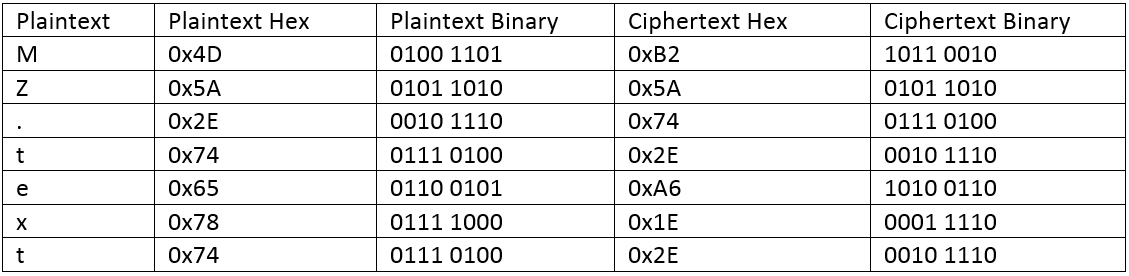What’s your finding here? Yes, the ciphertext only reverse the bit order of the plaintext! Now we can write a Python script to decrypt the file:

```def bit_reverse(byte):
out = 0
for i in range(0, 8):
out += (byte & 0x01)
out = (out << 1)
byte = (byte >> 1)
out = (out >> 1)
return out

data = ''
data += chr(bit_reverse(ord(ch)))

open('gdssagh.out.exe', 'wb').write(data)
print 'Result written to file gdssagh.out.exe.'
```

And run the decrypted file we can get the email address: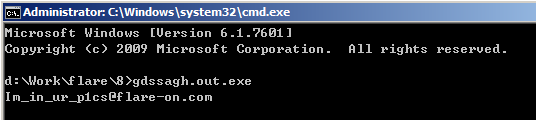Posted in CTF | Tagged , , , | Comments Off on FLARE On Challenge (2015) #8

## FLARE On Challenge (2015) #7

This challenge is a .NET application. There are many tools to decompile a .NET application and here I use the ILSpy.

A quick look at the decompile result I found that this application is probably obfuscated by SmartAssembly :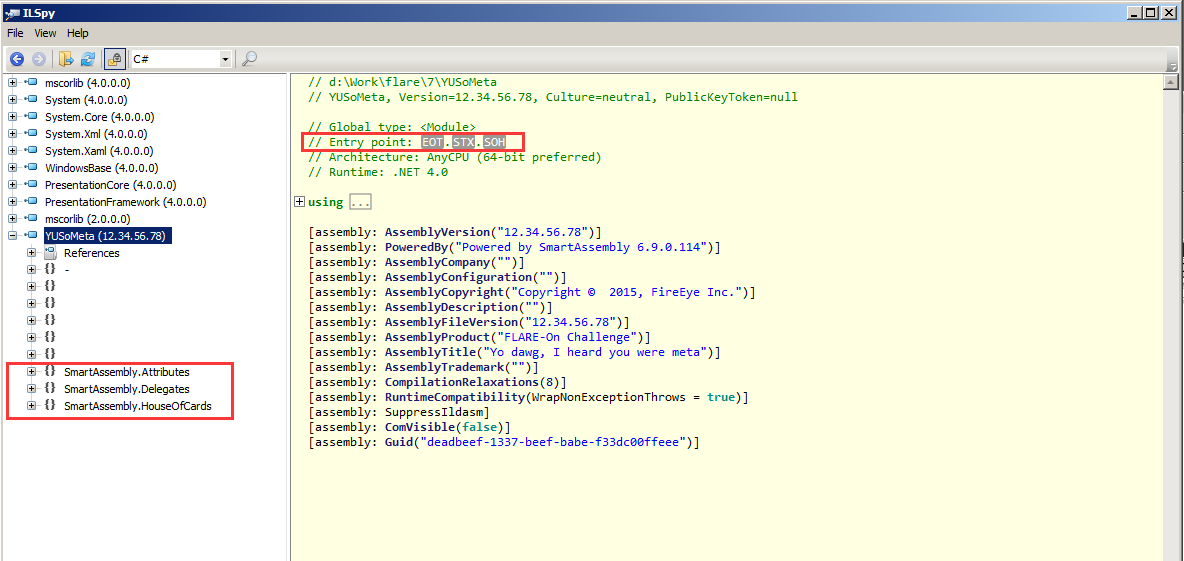There is a powerful tool named d4dot which can help with the deobfuscation. The tool is easy to use so I will not talk about more details here. After the deobfuscation the code looks much better and let’s go to the Entry Point function at first: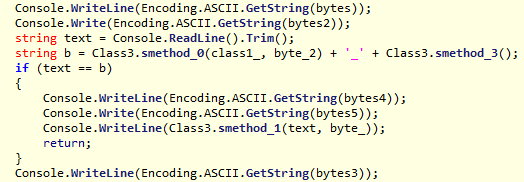This function is easy to understand, and the most important logic here is to compare your input with a string stored in a variable named “b”. And the string “b” is made up with two parts that generated by two different functions Class3.smethod_0() and Class3.smethod_3(). The code of Class3.smethod_0() is shown as below: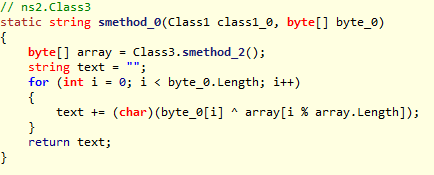This function does a simple XOR calculation on the array returned by function Class3.smethod_2() and the second arguments passed to this function. Here is the code of function Class3.smethod_2():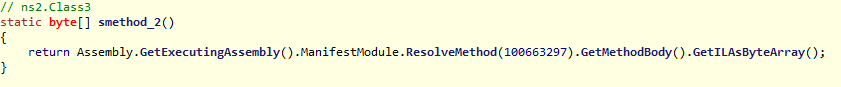This function is interesting, it returns the byte code of method 100663297 as a byte array. So what is method 100663297? If you are not familiar with .NET file format, I recommend you to read the article here at first:
http://www.codeproject.com/Articles/12585/The-NET-File-Format

In short, the 100663297 (0x06000001) is a .NET token which can be split into two parts, the highest 8 bit (0x06) is an index for a MetaData table, and the rest 24 bits (0x000001) is the index of the item in that table. Following is a list of .NET MetaData tables: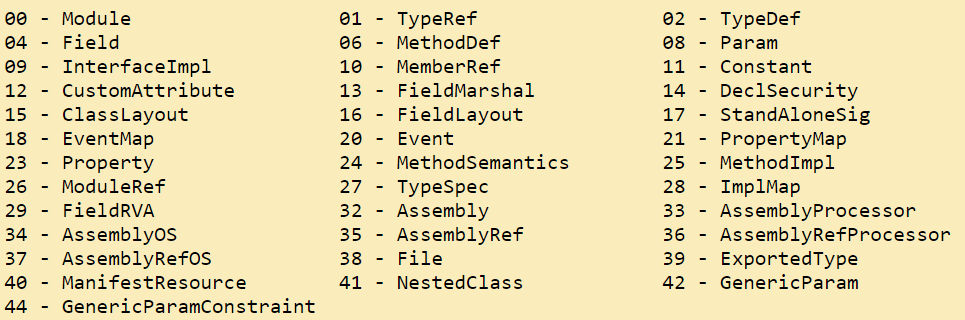The index 0x06 here represents the MethodDef table. We can use the CFF explorer to view the .NET tables. With this tool we can find that the first item (0x000001) in the MethodDef table is a function named .cctor, and we can also disassemble this function: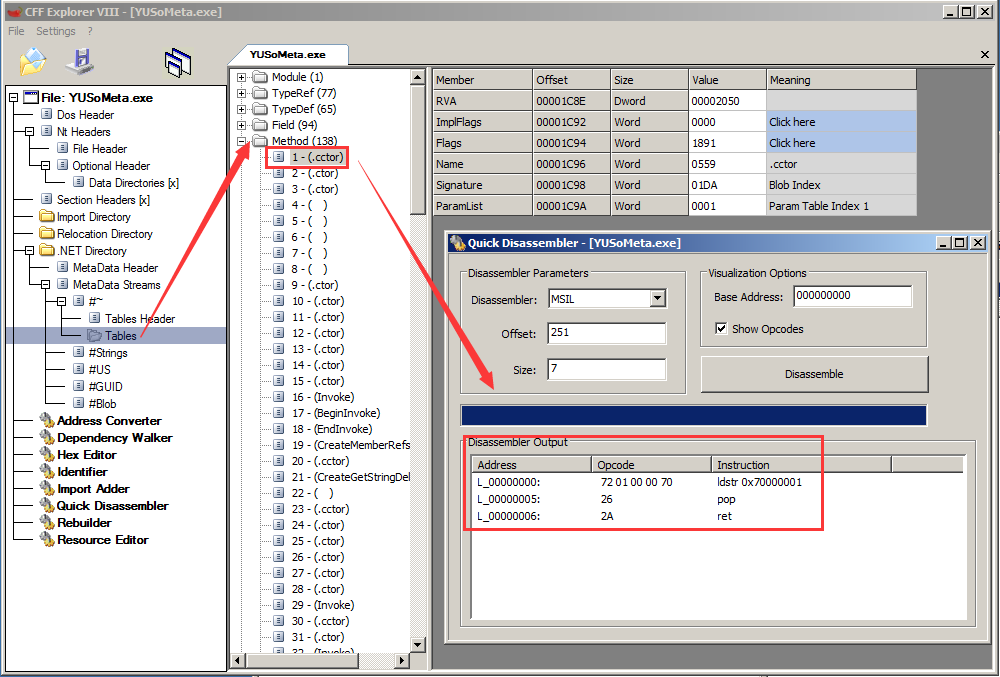Now that we have the byte code of the function, and we also know the second augments passed to the Class3.smethod_0() (from the decompiled code), we can easily calculate the first part of the string “b”:

```bytecode =(0x72, 0x01, 0x00, 0x00, 0x70, 0x26, 0x2A)
arg2 = (31, 100, 116, 97, 0, 84, 69, 21, 115, 97, 109, 29, 79, 68, 21, 104, 115, 104, 21, 84, 78)

bytecode_len = len(bytecode)
out = ''
i = 0

for b in arg2:
out += chr(b ^ bytecode[i%bytecode_len])
i+= 1

print out
```

Ok, now we can move to the second part of string “b”, which is generated by function Class3.smethod_3():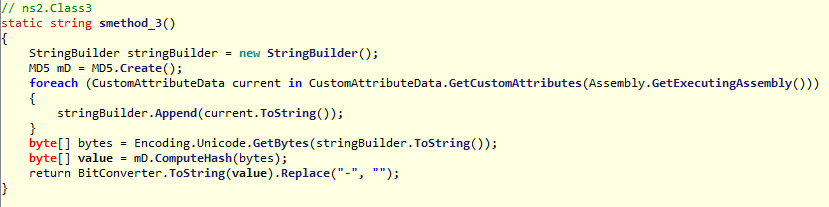This function also straightforward, it concatenates all the CustomAttributeData as a string and then calculates the MD5 hash of it. The CustomAttributeData can be easily read from the .NET decompiler: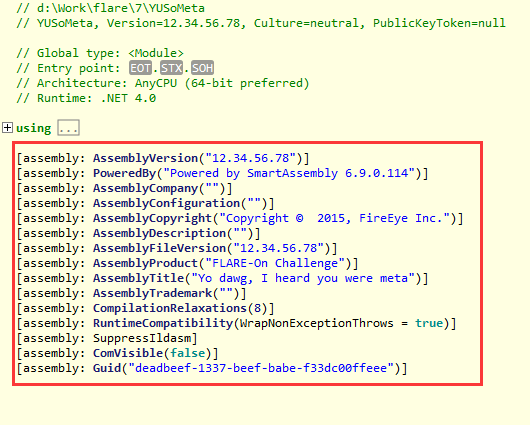However, this is a problem that we don’t know the correct order to put those CustomAttributeData together as a string. Since there are not too many CustomAttributeData, one possible way is to brute-force all the orders. But I will not solve this challenge in this way.

As we already have the first part of the string “b”, when this program compares the string with our input, the string must present in the memory, so why not just search it in the memory?

With this idea I launched this program in a debugger and let it run directly. It will ask us to enter a password: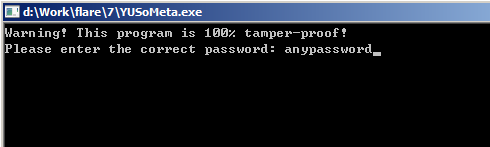We can input any password and continue executing the program until it exits. Now open the memory window and search for the first part of the string in Unicode format: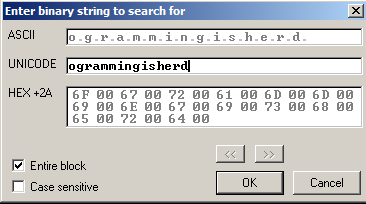And finally we will  get what we want in the memory: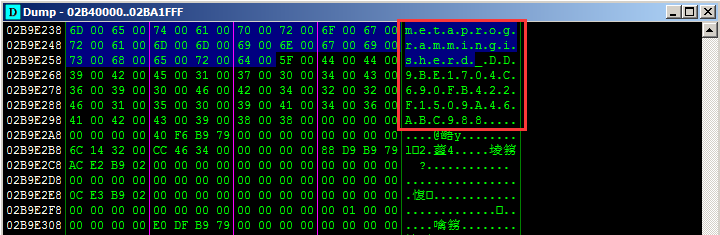Provide the correct password to this program we have the email address: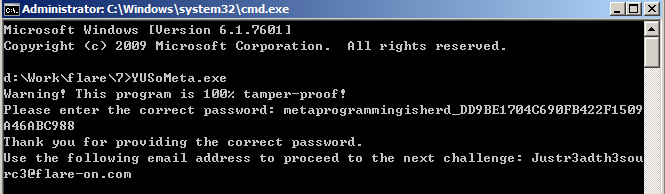Posted in CTF | Tagged , , , | Comments Off on FLARE On Challenge (2015) #7

## FLARE On Challenge (2015) #6

This challenge is an Android application. There are a lot of tools can be used to analyze Android application and the JEB Decompiler is my favorite one. Let’s open this challenge in JEB and look at the Manifest file at first: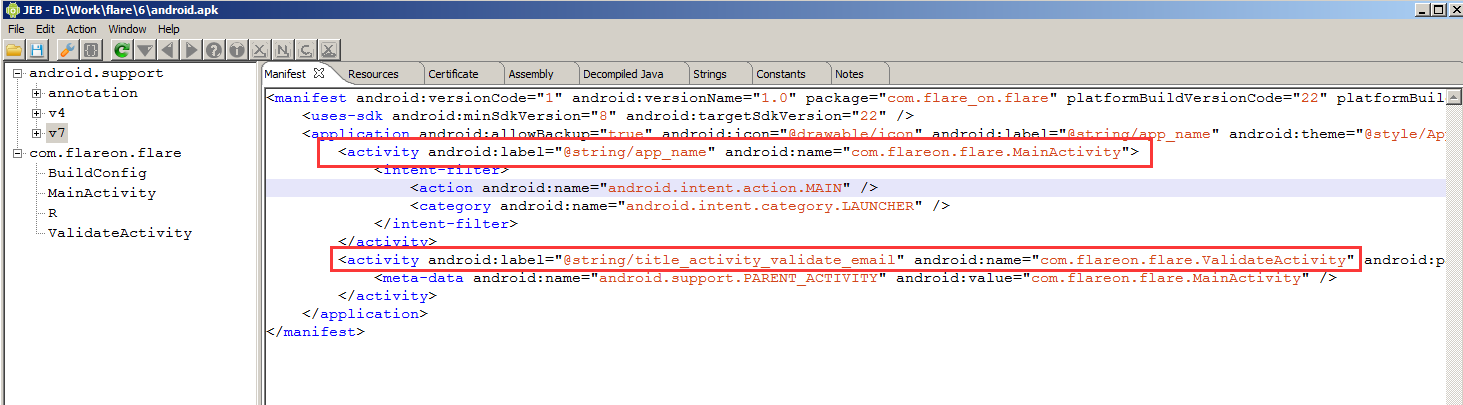The Manifest gives us many information and the most important are the two Activity: com.flareon.flare.MainActivity and com.flareon.flare.ValidateActivity.

Let’s see what will the MainActivity do: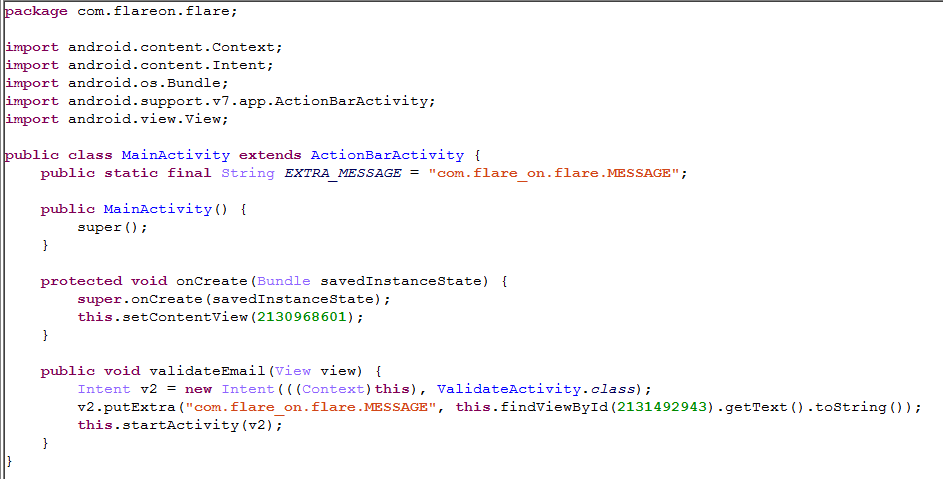The functionality looks simple: it reads the input and stores it as an extra data named com.flare_on.flare.MESSAGE, and then it launches the ValidateActivity. So we move to the ValidateActivity: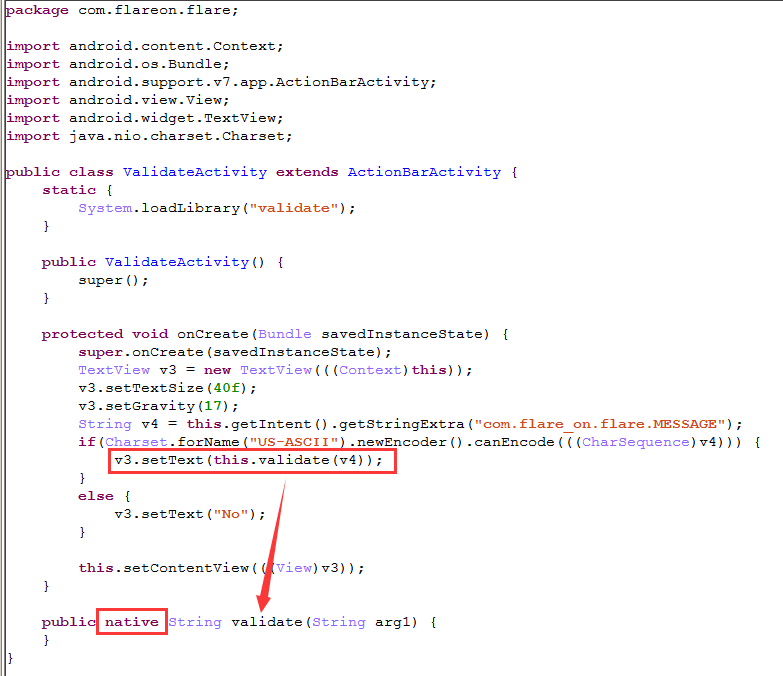The ValidateActivity will retrieve the extra data stored in the com.flare_on.flare.MESSAGE, then verify if it is an ASCII string, if so it will pass this string to a function named “validate”, if not it will set the text “No”. So what does the function validate() do? From the keyword “native” in the function definition we can know that this is actually a native function which could exist in a native library. If you decompress the APK file with a decompression tool like 7-zip you may notice that there is a folder named lib and inside this folder there is an interesting file named libvalidate.so, from the file name we can guess that this one should be the file we want. So let’s disassemble it in IDA. From the Entry Point List (Ctrl + E) we can see a function named Java_com_flareon_flare_ValidateActivity_validate, which is interesting: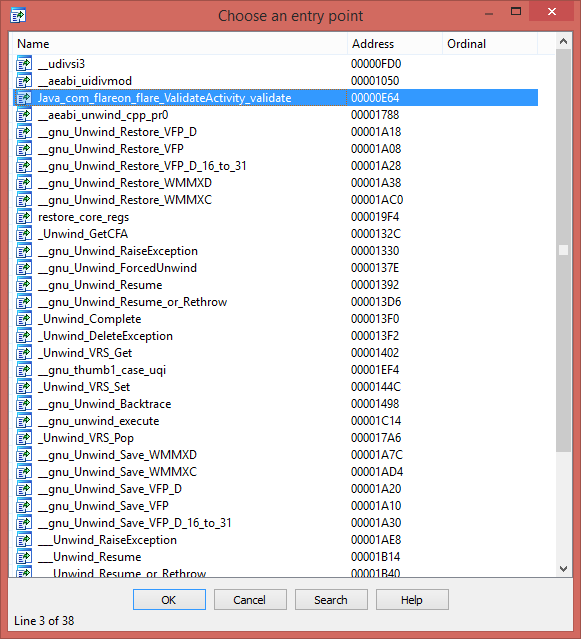A quick navigate on this function we can find that there are two strings “That’s it!” and “No” present at the end of this function: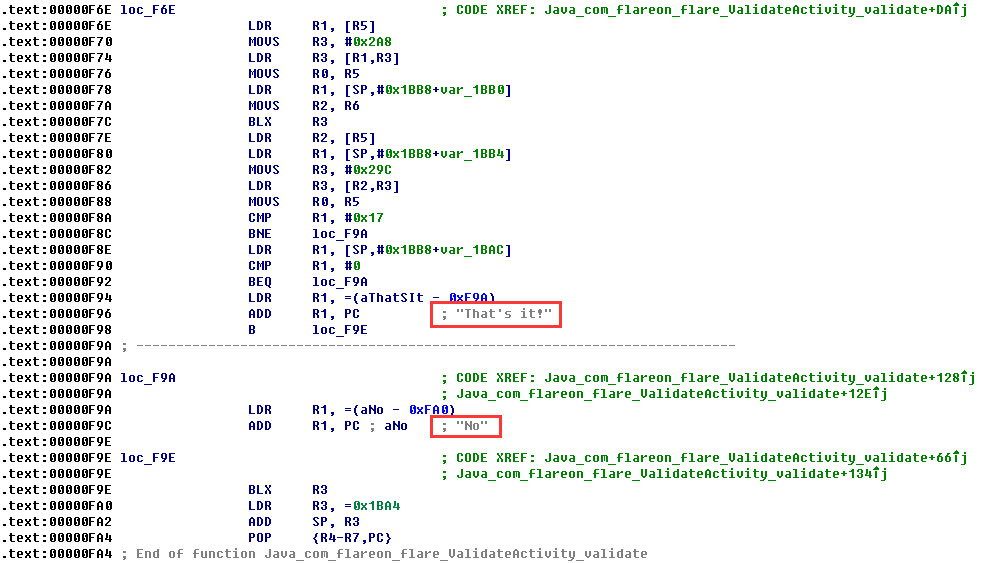Those strings confirm that this function is what we need, so the next thing to do is no more than read the disassembly code to understand the functionality of this function. In short, this function will verify if the length of the input string is equal to or less than 46 at first, then it will convert two adjacent bytes of the input string to a 16-bit WORD (e.g. if two adjacent bytes are 0x11 and 0x22, they will be converted to 0x1122), and next, it will calculate the remainder of the WORD by dividing each 16-bit WORD in a table (I call it mod_table) located at address 0x00002214, if the remainder is zero, it will increase the relevant byte in another table (I call it reminder_table) by 1, and use the dividing result as a new DWORD to calculate the remainder until the dividing result equal to or less than 1. And finally it will compare the reminder_table with a hard-coded table (I call it key_table) to see if they are match. The following pseudo code may help you understand the flow:

```if (input.length > 46)
return "No"

result = 0
for (i = 0, table_index = 0; i <= input.length; i += 2, table_index++)
{
n = (input[i] << 8) | input[i+1]

j = 0
while (j < 0xD94)
{
while ((n % mod_table[j]) == 0)
{
reminder_table[table_index][j] += 1
n = n / mod_table[j]
if (n <= 1)
goto label_next
}
j++
}
label_next:
if (reminder_table[table_index] == key_table[table_index])
{
result += 1
}
}

if (result == 0x17)
return "That's it!"

return "No"
```

Obviously, this algorithm can be easily reversed, the following IDA Python script can be used to calculate the correct input:

```from idaapi import *
import struct

mod_table = 0x00002214
key_tables = 0x00005004
key_tables_len = 0x17
key_table_len = 0xD94
out_str = ''

for i in range(0, key_tables_len):
key_table = Dword(key_tables + i*4)
out = 1
for j in range(0, key_table_len):
index = Word(key_table + j*2)
if index != 0:
num = Word(mod_table + j*2)
out = out * (num ** index)
out_str += struct.pack('<H', out)[::-1]

print out_str
```
Posted in CTF | Tagged , , , | Comments Off on FLARE On Challenge (2015) #6